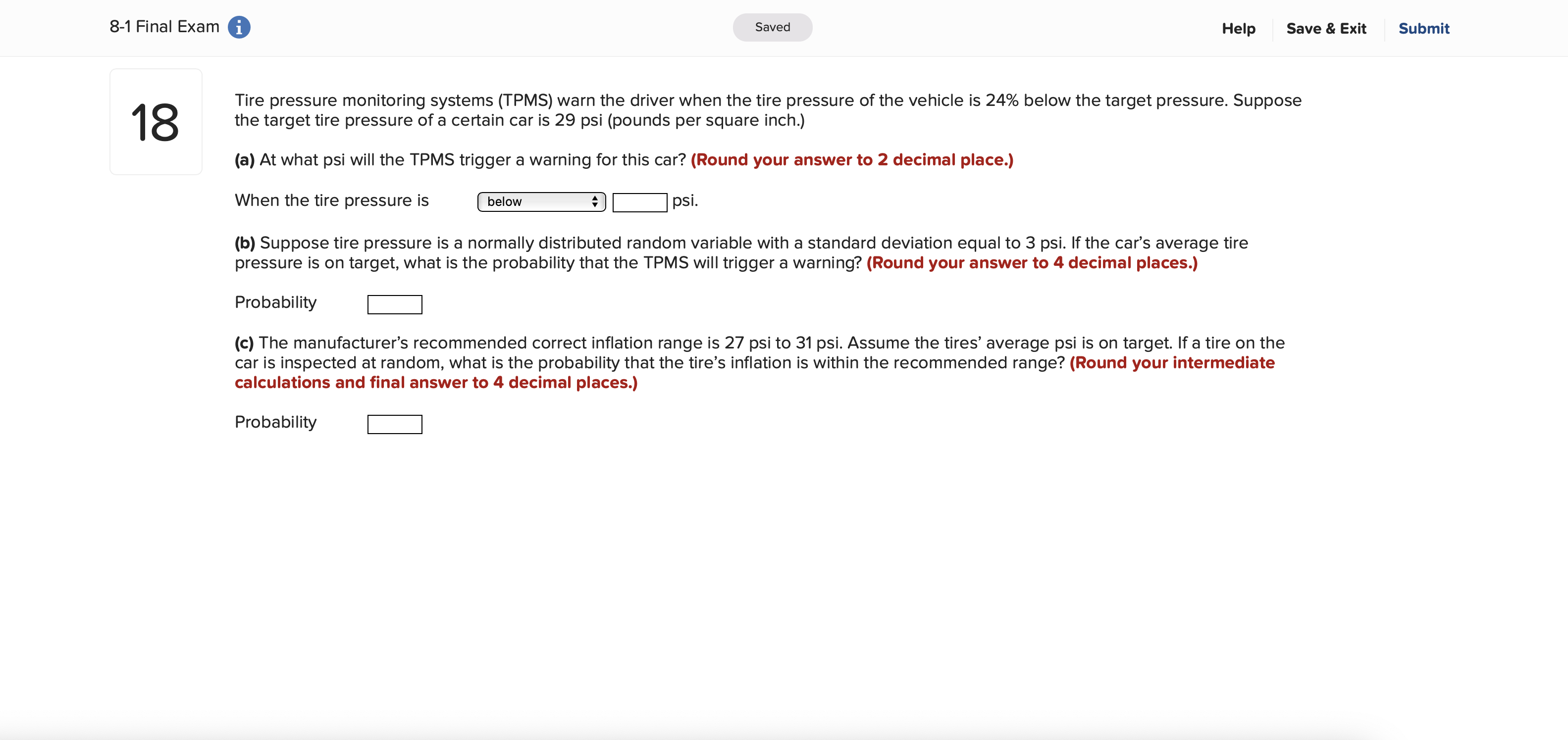# Tire pressure monitoring systems (TPMS) warn the driver when the tire pressure of the vehicle is 24% below the target pressure. Suppose the target tire pressure of a certain car is 29 psi (pounds per square inch.) (a) At what psi will the TPMS trigger a warning for this car? (Round your answer to 2 decimal place.) When the tire pressure is below psi. (b) Suppose tire pressure is a normally distributed random variable with a standard deviation equal to 3 psi. If the car's average tire pressure is on target, what is the probability that the TPMS will trigger a warning? (Round your answer to 4 decimal places.) Probability (c) The manufacturer's recommended correct inflation range is 27 psi to 31 psi. Assume the tires' average psi is on target. If a tire on the car is inspected at random, what is the probability that the tire's inflation is within the recommended range? (Round your intermediate calculations and final answer to 4 decimal places.) Probability

Questionhelp_outlineImage TranscriptioncloseTire pressure monitoring systems (TPMS) warn the driver when the tire pressure of the vehicle is 24% below the target pressure. Suppose the target tire pressure of a certain car is 29 psi (pounds per square inch.) (a) At what psi will the TPMS trigger a warning for this car? (Round your answer to 2 decimal place.) When the tire pressure is below psi. (b) Suppose tire pressure is a normally distributed random variable with a standard deviation equal to 3 psi. If the car's average tire pressure is on target, what is the probability that the TPMS will trigger a warning? (Round your answer to 4 decimal places.) Probability (c) The manufacturer's recommended correct inflation range is 27 psi to 31 psi. Assume the tires' average psi is on target. If a tire on the car is inspected at random, what is the probability that the tire's inflation is within the recommended range? (Round your intermediate calculations and final answer to 4 decimal places.) Probability fullscreen

### Want to see this answer and more?

Experts are waiting 24/7 to provide step-by-step solutions in as fast as 30 minutes!*

*Response times may vary by subject and question complexity. Median response time is 34 minutes for paid subscribers and may be longer for promotional offers.
Tagged in
Math